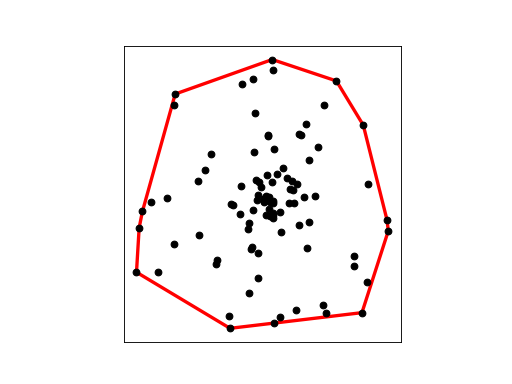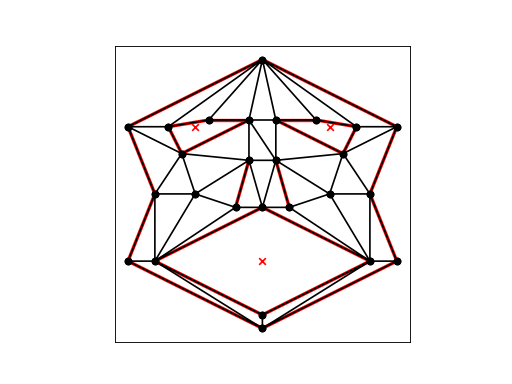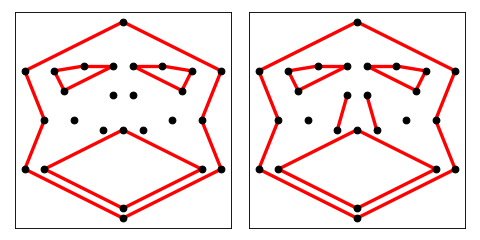# Convex Hulls and Mesh Boundaries¶

If the input is a vertex set (rather than a PSLG), Triangle produces its convex hull as a by-product in the output’s segments if you use the -c switch. There are faster algorithms for finding a two-dimensional convex hull than triangulation, of course, but this one comes for free. In the example below, the data dots is read, and its convex hull produced in dots.1.If the input is an unconstrained mesh (you are using the -r switch but not the -p switch), Triangle produces a list of its boundary edges (including hole boundaries) as a by-product when you use the -c switch. If you also use the -p switch, the output will contain all the segments from the input as well. For example, consider the mesh described by face.1.```triangulate(get_data('face.1'), 'rc')
triangulate(get_data('face.1'), 'rpc')
```

In each case, the boundary segments can be found in face.2.poly.